# How to Show formula but not result in Excel

Excel performs a function in a cell when using equals to sign (=).
For example, if you type =2+2 in a cell it will result in 4.

Excel lets you view formulas with the following given methods.

1. Via Show Formulas option
2. Using single quotation mark ( ‘ ) in front of the formula

Let’s understand how to show formulas only in Excel.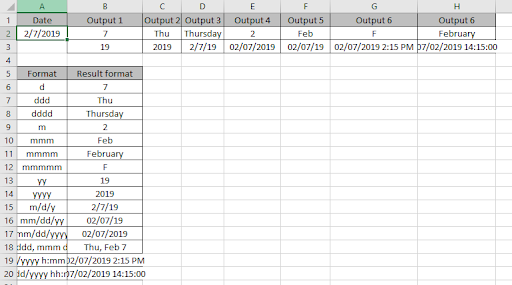Here we have some data where results have been extracted using Excel formulas.

Go to tab Formulas > Show formulas under Formula Auditing.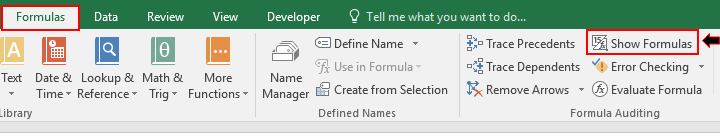Click Show Formula option to view all formulas of the sheet.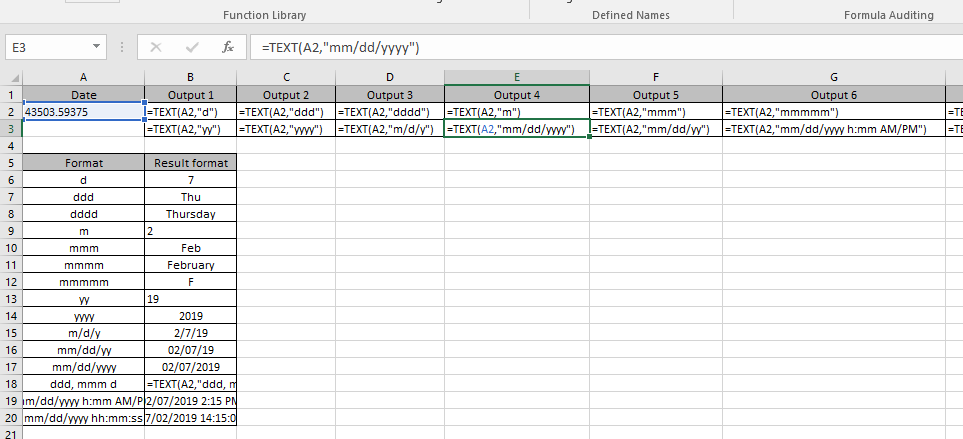This option lets you view all Excel Formulas used in the worksheet instead of the results.
There’s one more way to view excel formulas, not the result. If you wish to view the Formula of a particular cell. Then use the below-mentioned method.

Single Quote mark in front the formula lets you show only formula not the result of the cell.

Here I have used this method on a Excel formula.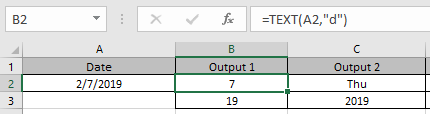Now I wish to view formulas on B2 & C3 cell here. Just apply one single quote mark in front of the formula.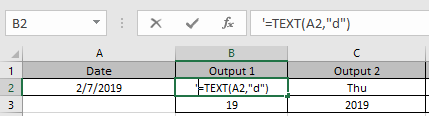Apply the same in C3 cell to view excel formula instead of results.As you can see in the above snapshot Excel formula not the result in the cell.

Hope You understood how to show Formulas instead of results in Excel. Explore more articles on Excel functions and Formulas here. Please state your queries in the comment box below. We will help you.

Related Articles:

Excel Shortcut Keys for Merge and Center

How to Delete only filtered Rows in Excel

How to Text to Time Conversion in Excel

How to Freeze Panes in Microsoft Excel

How to Install Analysis ToolPak in Excel For Mac and Windows

Popular Articles:

50 Excel Shortcuts to Increase Your Productivity

How to use the VLOOKUP Function in Excel

How to use the COUNTIF function in Excel

How to use the SUMIF Function in Excel

Terms and Conditions of use

The applications/code on this site are distributed as is and without warranties or liability. In no event shall the owner of the copyrights, or the authors of the applications/code be liable for any loss of profit, any problems or any damage resulting from the use or evaluation of the applications/code.